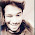### Deepak's blog

there are three variants of gradient descent,which differ in how much data we use to compute the gradient of objective function Depending on the amount of data , we make a trade-off between the accuracy of the parameter update and the time it takes to perform as update

### In code, batch  gradient descent looks like this:

for i in range(nd_epochs):
params_grad = eval uate_gra dient ( loss_function , data , params )
params = params - learning_rate * params_grad

### now lets look Gradient Descent ..

When you venture into machine learning one of the fundamental aspects of your learning would be to understand “Gradient Descent”. Gradient descent is the backbone of an machine learning algorithm. In this article I am going to attempt to explain the fundamentals of gradient descent using python code. Once you get hold of gradient descent things start to be more clear and it is easy to understand different algorithms.Much has been already written on this topic so it is not going to be a ground breaking one.  you will need some basic python packages viz. numpy and matplotlib to visualize.
Let us start with some data, even better let us create some data. We will create a linear data with some random Gaussian noise.
X = 2 * np.random.rand(100,1)
y = 4 +3 * X+np.random.randn(100,1)
Next let’s visualize the data
you may study that line can be expressed as:

And then you can solve the equation for b and m as follows:

To explain in brief about gradient descent, imagine that you are on a mountain and are blindfolded and your task is to come down from the mountain to the flat land without assistance. The only assistance you have is a gadget which tells you the height from sea-level. What would be your approach be. You would start to descend in some random direction and then ask the gadget what is the height now. If the gadget tells you that height and it is more than the initial height then you know you started in wrong direction. You change the direction and repeat the process. This way in many iterations finally you successfully descend down.
Well here is the analogy with machine learning terms now:
Size of Steps took in any direction = Learning rate
Gadget tells you height = Cost function
Looks simple but mathematically how can we represent this. Here is the maths:
where m = number of observation
this is an example of linear regression

I am taking an example of linear regression.You start with a random Theta vector and predict the h(Theta), then derive cost using the above equation which stands for Mean Squared Error.  The partial derivative is something that can help to find the Theta for next iteration

But ,what if we had multiple features then we would have multiple Theta. Don’t worry here is a generalized form to calculate Theta:
where alpha = Learning Rate
if alpha is too small,gradient descent can be slow . if alpha is too large ,gradient descent can overshoot the minimum.it may fail to converge,or even diverge .

1.Excellent mind blowing

2.Deepak thopu dammu vuntey aapu

3.Pretty good to see the explanation Go ahead and Make sure U r in the right direction 😉

4.Excellent bro... Will u teach me this!!

1.Yeah sure, ...!